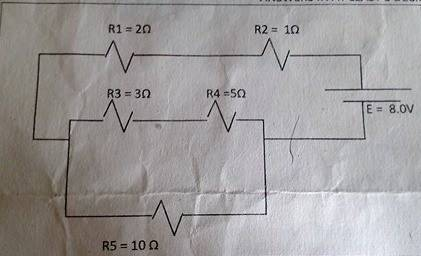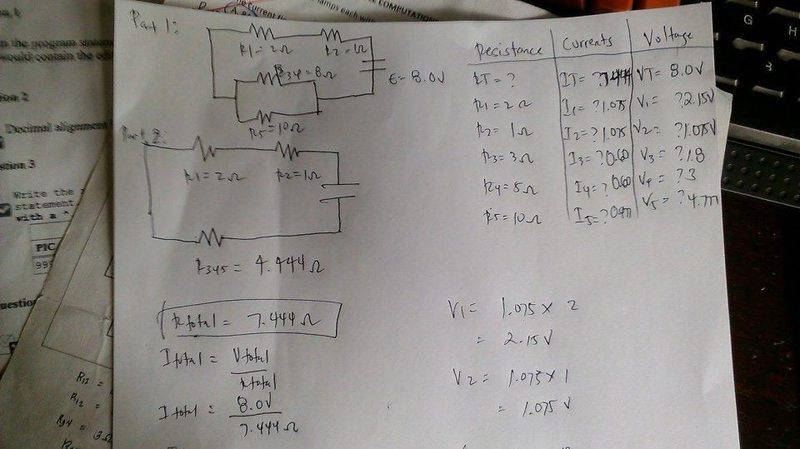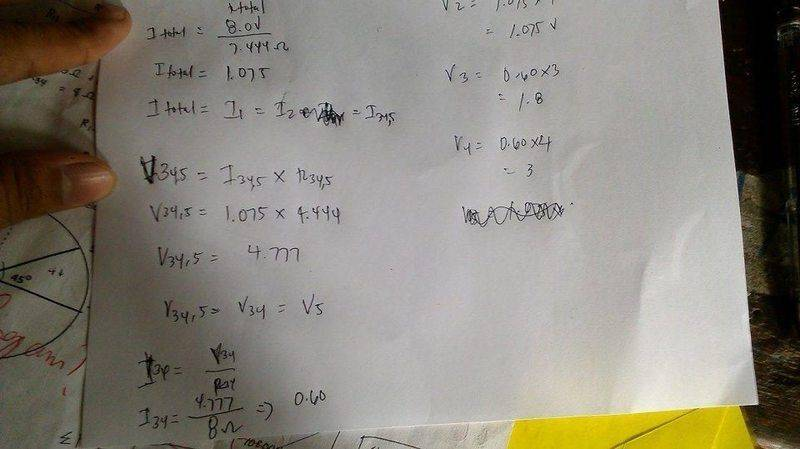# Series and Parallel Combination

[Note: Thread has been moved to the homework forums by a mentor]

This is the Given problemThis is my solution part 1
- What I did here is I series the R3 and R4 (R3 + R4), and I parallel the R34 to R5 (most of the calculation are from the calculator)This is my solution part 2The problem is when I check and compute the total voltage from V1,V2,V3,V4,V5 the result is 12 and more volts not 8 volts exactly, I cant locate my error and miscalculation

SteamKing
Staff Emeritus
Homework Helper
[Note: Thread has been moved to the homework forums by a mentor]

This is the Given problem
View attachment 100290
This is my solution part 1
- What I did here is I series the R3 and R4 (R3 + R4), and I parallel the R34 to R5 (most of the calculation are from the calculator)
View attachment 100292

This is my solution part 2

View attachment 100293

The problem is when I check and compute the total voltage from V1,V2,V3,V4,V5 the result is 12 and more volts not 8 volts exactly, I cant locate my error and miscalculation
The problem comes when you check the voltage drops in the parallel resistor section. In the parallel resistors, the current of 1.075A divides, so there is a different current in each branch. The same voltage drop, however, is present in each branch.

For this circuit, I5 ⋅ 10 Ω = I34 ⋅ (3 Ω + 5 Ω), and I34 + I5 = 1.075 A

The problem comes when you check the voltage drops in the parallel resistor section. In the parallel resistors, the current of 1.075A divides, so there is a different current in each branch. The same voltage drop, however, is present in each branch.

For this circuit, I5 ⋅ 10 Ω = I34 ⋅ (3 Ω + 5 Ω), and I34 + I5 = 1.075 A

Yes, The current in I3 and I4 is different to I5, since I34 is parallel to I5 their voltage is the same. Sorry I still don't get it, maybe I'm missing something. My circuit in I3 and I4 is .60 and in I5 is .477 so to add = 1.077A which is close.

SteamKing
Staff Emeritus
Homework Helper
Yes, The current in I3 and I4 is different to I5, since I34 is parallel to I5 their voltage is the same. Sorry I still don't get it, maybe I'm missing something. My circuit in I3 and I4 is .60 and in I5 is .477 so to add = 1.077A which is close.
In your original voltage check, you are adding the voltage drop from both branches of the parallel resistors. The voltage drop in each branch is the same; you only need to add this voltage drop once to the drops in R1 and R2.

In your original voltage check, you are adding the voltage drop from both branches of the parallel resistors. The voltage drop in each branch is the same; you only need to add this voltage drop once to the drops in R1 and R2.

Sorry, But I still don't get it, what must be the V5, V3 and V4 should be?

SteamKing
Staff Emeritus
Homework Helper
Sorry, But I still don't get it, what must be the V5, V3 and V4 should be?
V5 = I5 ⋅ 10Ω

I think you can work out what V3 and V4 are.

The point is in your original voltage drop calculations around the circuit, you counted the voltage drop in each branch of the parallel resistors separately, which is why you got a total voltage drop of more than 8 volts. You only need to count the voltage drop in one branch of the parallel resistors, either V5 or V3 + V4, when checking that the voltage drop in the circuit balances the voltage from the battery.

V5 = I5 ⋅ 10Ω

I think you can work out what V3 and V4 are.

The point is in your original voltage drop calculations around the circuit, you counted the voltage drop in each branch of the parallel resistors separately, which is why you got a total voltage drop of more than 8 volts. You only need to count the voltage drop in one branch of the parallel resistors, either V5 or V3 + V4, when checking that the voltage drop in the circuit balances the voltage from the battery.

Meaning Vtotal = V1 + V2 + V3 + V4 OR V1 + V2 + V5?

SteamKing
Staff Emeritus